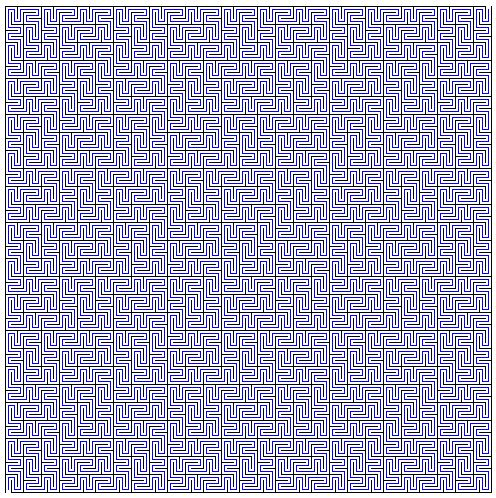# Plane Filling Curves: Peano's & Wunderlich's

On another occasion I mentioned that Peano's definition of his plane filling curve was entirely analytic. It could be shown [Sagan, p. 36], however, that Peano's original curve could be obtain geometrically as the limit of a sequence of curves as was the case with Hilbert's curve. The difference between Peano's and Hilbert's constructions is that Hilbert maps intervals of length 2-2n into squares of size 2-n×2-n, whereas Peano's construction is equivalent to mapping intervals of length 3-2n into squares of size 3-n×3-n.

Walter Wunderlich [Sagan, p. 45] constructed several modifications of Peano curves. The applet below demonstrates the (equivalent) Peano construction and three Wunderlich's curves. Wunderlich characterized all possible plane filling curves whose construction requires mapping intervals of length 3-2n into squares of size 3-n×3-n as being of two types: switch-back (Peano and the first two curves) and meandering (the third curve.)

(Keep clicking in the applet.)

### If you are reading this, your browser is not set to run Java applets. Try IE11 or Safari and declare the site https://www.cut-the-knot.org as trusted in the Java setup.What if applet does not run?

There are only two curves of the meandering type. They are reflections of each other in the (0,0)-(1,1) diagonal.

The number of switch-backs is significantly larger. Starting basically with the same S shape, on the next step, in each of the nine available squares one has to select one of two possible alternatives, which leads to the estimate of 29 = 512 curves defined by 9-digit binary sequences. Of these, 24·2 = 32 are palindromic and lead to curves with central symmetry. The remaining asymmetric 480 = 512 - 32 split into pairs, in each of which the curves are obtained from each other by central symmetry with respect to the center of the unit square. Therefore, there appears to be 480/2 + 32 = 272 essentially distinct "Peano" curves.### Peano's Definition

The original Peano curve maps the unit interval [0,1] onto the unit square [0,1]×[0,1]. The mapping is based on the ternary system, so that all digits t below may only take on values 0, 1, 2. First define the digit transformation k:

kt = 2 - t.

Peano's function fp maps a ternary fraction .t1t2t3 ... into a point (xp(.t1t2t3 ...), yp(.t1t2t3 ...)) in the unit square, where xp and yp are defined, respectively, as

xp(.t1t2t3 ...) = .t1(kt2t3)(kt2+t4t5) ...
yp(.t1t2t3 ...) = .(kt1t2)(kt1+t3t4) ...

Where kv denotes, as usual, the vth iterate of k.

### Reference

1. H. Sagan, Space-Filling Curves, Springer-Verlag, 1994### Plane Filling Curves•